# Histogram Equalization¶

## Goal¶

In this tutorial you will learn:

• What an image histogram is and why it is useful
• To equalize histograms of images by using the OpenCV function:equalize_hist:equalizeHist <>

## Theory¶

### What is an Image Histogram?¶

• It is a graphical representation of the intensity distribution of an image.
• It quantifies the number of pixels for each intensity value considered.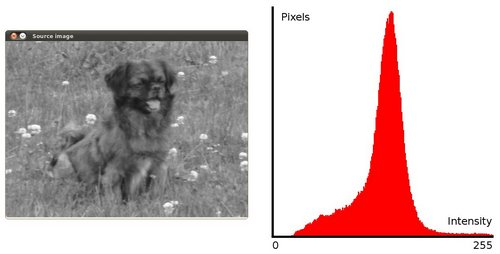### What is Histogram Equalization?¶

• It is a method that improves the contrast in an image, in order to stretch out the intensity range.
• To make it clearer, from the image above, you can see that the pixels seem clustered around the middle of the available range of intensities. What Histogram Equalization does is to stretch out this range. Take a look at the figure below: The green circles indicate the underpopulated intensities. After applying the equalization, we get an histogram like the figure in the center. The resulting image is shown in the picture at right.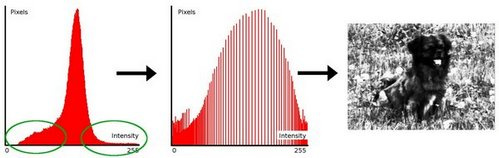### How does it work?¶

• Equalization implies mapping one distribution (the given histogram) to another distribution (a wider and more uniform distribution of intensity values) so the intensity values are spreaded over the whole range.

• To accomplish the equalization effect, the remapping should be the cumulative distribution function (cdf) (more details, refer to Learning OpenCV). For the histogram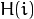, its cumulative distribution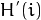is: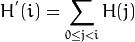To use this as a remapping function, we have to normalizesuch that the maximum value is 255 ( or the maximum value for the intensity of the image ). From the example above, the cumulative function is: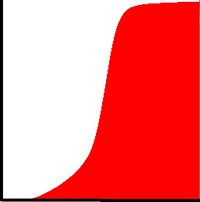• Finally, we use a simple remapping procedure to obtain the intensity values of the equalized image: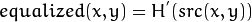## Code¶

• What does this program do?

• Convert the original image to grayscale
• Equalize the Histogram by using the OpenCV function EqualizeHist
• Display the source and equalized images in a window.

• Code at glance:

#include "opencv2/highgui.hpp"
#include "opencv2/imgproc.hpp"
#include <iostream>
#include <stdio.h>

using namespace cv;
using namespace std;

/**  @function main */
int main( int argc, char** argv )
{
Mat src, dst;

char* source_window = "Source image";
char* equalized_window = "Equalized Image";

src = imread( argv, 1 );

if( !src.data )
{ cout<<"Usage: ./Histogram_Demo <path_to_image>"<<endl;
return -1;}

/// Convert to grayscale
cvtColor( src, src, CV_BGR2GRAY );

/// Apply Histogram Equalization
equalizeHist( src, dst );

/// Display results
namedWindow( source_window, CV_WINDOW_AUTOSIZE );
namedWindow( equalized_window, CV_WINDOW_AUTOSIZE );

imshow( source_window, src );
imshow( equalized_window, dst );

/// Wait until user exits the program
waitKey(0);

return 0;
}


## Explanation¶

1. Declare the source and destination images as well as the windows names:

Mat src, dst;

char* source_window = "Source image";
char* equalized_window = "Equalized Image";


src = imread( argv, 1 );

if( !src.data )
{ cout<<"Usage: ./Histogram_Demo <path_to_image>"<<endl;
return -1;}

3. Convert it to grayscale:

cvtColor( src, src, CV_BGR2GRAY );

4. Apply histogram equalization with the function equalizeHist :

equalizeHist( src, dst );


As it can be easily seen, the only arguments are the original image and the output (equalized) image.

5. Display both images (original and equalized) :

namedWindow( source_window, CV_WINDOW_AUTOSIZE );
namedWindow( equalized_window, CV_WINDOW_AUTOSIZE );

imshow( source_window, src );
imshow( equalized_window, dst );

6. Wait until user exists the program

waitKey(0);
return 0;


## Results¶

1. To appreciate better the results of equalization, let’s introduce an image with not much contrast, such as:which, by the way, has this histogram: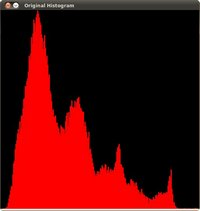notice that the pixels are clustered around the center of the histogram.

2. After applying the equalization with our program, we get this result: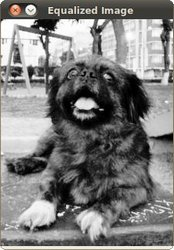this image has certainly more contrast. Check out its new histogram like this: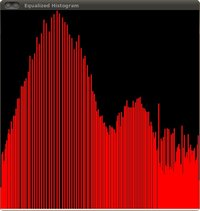Notice how the number of pixels is more distributed through the intensity range.

Note

Are you wondering how did we draw the Histogram figures shown above? Check out the following tutorial!